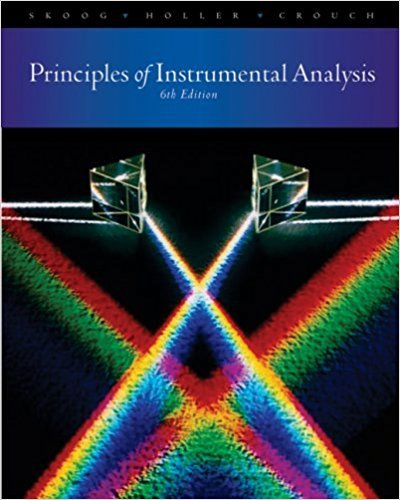×
×

# Aluminum forms a I: I complex with 2-hydroxy-lnaphthaldehydeISBN: 9780495012016 317

## Solution for problem 14-14 Chapter 14

Principles of Instrumental Analysis | 6th Edition

• Textbook Solutions
• 2901 Step-by-step solutions solved by professors and subject experts
• Get 24/7 help from StudySoup virtual teaching assistantsPrinciples of Instrumental Analysis | 6th Edition

4 5 1 324 Reviews
29
1
Problem 14-14

Aluminum forms a I: I complex with 2-hydroxy-lnaphthaldehyde p-methoxyhenzovlhvdraxonal.which absorbs UV radiation at 285 nm. Under pseudo-firstorder~o~ditions, a plot of the initial rate of the reaction (absorbance units persecond) versus the concentration of aluminum (in J.lM)yields a straight line describedby the equationrate = 1.74c" - 0.225Find the concentration of aluminum in a solution that exhibits a rate of 0.76 absorbanceunits per second under the same experimental conditions.

Step-by-Step Solution:
Step 1 of 3

Calculations with Ka & Kb Values • for a conjugate acid-base pair, Ka x Kb = Kw, where Kw = 1.0 x 10^-14 at 25˚C ex. Is a solution of NH4HC2O4 at 25˚C acidic, basic, or neutral • Ka C2H2O4 = 5.6 x 10^-2 Kb NH3 = 1.8 x 10^-5 Solve for new Ka for NH4+ + H2O⁶NH3 + H3O+ Ka* = 1.0 x 10^-14 = 5.56 x 10^-10...

Step 2 of 3

Step 3 of 3

##### ISBN: 9780495012016

Since the solution to 14-14 from 14 chapter was answered, more than 218 students have viewed the full step-by-step answer. The full step-by-step solution to problem: 14-14 from chapter: 14 was answered by , our top Chemistry solution expert on 03/02/18, 06:21PM. This full solution covers the following key subjects: . This expansive textbook survival guide covers 34 chapters, and 619 solutions. This textbook survival guide was created for the textbook: Principles of Instrumental Analysis , edition: 6. Principles of Instrumental Analysis was written by and is associated to the ISBN: 9780495012016. The answer to “Aluminum forms a I: I complex with 2-hydroxy-lnaphthaldehyde p-methoxyhenzovlhvdraxonal.which absorbs UV radiation at 285 nm. Under pseudo-firstorder~o~ditions, a plot of the initial rate of the reaction (absorbance units persecond) versus the concentration of aluminum (in J.lM)yields a straight line describedby the equationrate = 1.74c" - 0.225Find the concentration of aluminum in a solution that exhibits a rate of 0.76 absorbanceunits per second under the same experimental conditions.” is broken down into a number of easy to follow steps, and 67 words.

Unlock Textbook Solution